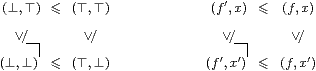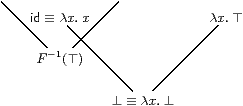## 4  The origins of the Euclidean principle

This characterisation of the powerset offers us a radically new attitude to the foundations of set theory: the notion of subset is a phenomenon in the macroscopic world that is a consequence of a purely algebraic (“microscopic”) principle, and is made manifest to us via the monadic assumption. Taking this point of view, where does the Euclidean principle itself come from?

Remark 4.1 The name was chosen to be provocative. The reason for it is not the geometry but the number theory in Euclid’s Elements, Book VII: with f,n∈Σ≡ ℕ, ⊤≡ 0 and ∧≡ hcf, we have a single step of the Euclidean algorithm,

 hcf(n,(f+n)) = hcf(n,(f+0)).

In fact, hcf(F(n),n) = hcf(F(0),n) for any polynomial F:ℕ→ℕ, since F(n)−F(0)=n× G(n) for some polynomial G. I do not know what connection, if any, this means that there is between higher order logic and number theory; the generalisation is not direct because ring homomorphisms need not preserve hcf, whereas lattice homomorphisms do preserve meets.

The way in which the Euclidean principle (and the Phoa principle in the next section) express F(σ) in terms of F(⊥) and F(⊤) is like a polynomial or power series (Taylor) expansion, except that it only has terms of degree 0 and 1 in σ, ∧ being idempotent. These principles may perhaps have a further generalisation, of which the Kock–Lawvere axiom in synthetic differential geometry [Koc81] would be another example. Of course, if ∧ is replaced by a non-idempotent multiplication in ΣX then the connection to the powerset is lost.

The Euclidean algorithm may be stated for any commutative ring, but it is by no means true of them all. But it is a theorem for the free (polynomial) ring in one variable, and we have similar results in logic, where ΣΣ is the free algebra (for the monad) on one generator. [See also the discussion in §O 7.7.]

Post-Publication Note. The following is only valid for terms whose free variables are of type ℕ or Σ. In particular, F cannot be a free variable.

Theorem 4.2 The Euclidean principle holds in the free model of the other axioms in Remark 3.8.

Proof    There is a standard way of expressing the free category with certain structure (including finite products) as a λ-calculus (with cut and weakening), in which the objects of the category are contexts, rather than types [Tay99, Chapter IV]. In our case, C has an exponentiating internal semilattice, and the calculus is the simply typed λ-calculus restricted as in Remark 2.5, together with the semilattice equations. The calculus gives the free category because the interpretation or denotation functor ⟦−⟧:CD is the unique structure-preserving functor to any other category of the same kind.

The free category C in which the adjunction Σ(−)⊣Σ(−) is monadic will be constructed (and given its own λ-calculus) in Section B 4, from which (for the present purposes) we only need to know that C(−,ΣΣ)≅C(−,ΣΣ).

So we just have to show that φ∧ F(φ∧ψ)⇔ φ∧ F(ψ) for all φ,ψ∈Σ, by structural induction on F∈ΣΣ in the appropriate λ-calculus, considering the cases where F(σ) is

1. ⊤ or another variable τ: trivial;
2. σ itself: by associativity and idempotence of ∧;
3. Gσ∧ Hσ: by associativity, commutativity and the induction hypothesis;
4. λ x.G(σ,x): using φ∧λ x.G(x,σ)=λ x.(φ∧ G(x,σ)) and the induction hypothesis;
5. G(H(σ),σ): put ψ′≡ Hψ and ψ″≡ H(φ∧ψ), so
 φ∧ψ′ ≡  φ∧ Hψ ⇔  φ∧ H(φ∧ψ) ≡  φ∧ψ″
by the induction hypothesis for H, and
 φ∧ G(Hψ,ψ) ≡ φ∧ G(ψ′,ψ) ⇔ φ∧ G(φ∧ψ′,φ∧ψ) ⇔ φ∧ G(φ∧ψ″,φ∧ψ) ⇔ φ∧ G(ψ″,φ∧ψ) ≡ φ∧ G(H(φ∧ψ),φ∧ψ)
by the induction hypothesis for (each argument of) G.         ▫

Remark 4.3 The result remains true for further logical structure, because of generalised distributivity laws:

1. ∨ and ⊥, assuming the (ordinary) distributive law;
2. ⇒, by an easy exercise in natural deduction;
3. ∃, assuming the Frobenius law;
4. ∀, since it commutes with φ∧(−);
5. ℕ, since φ∧rec(n,G(n),λ mτ.H(n,m,τ)) ⇔   rec(n,φ∧ G(n),λ mτ.φ∧ H(n,m,τ));
6. = and ≠, since these are independent of the logical variable;
7. Scott continuity, as this is just an extra equation (Remark 7.11) on the free structure, but Corollary 5.5 proves the dual Euclidean principle more directly in this case.         ▫

Remark 4.4 Todd Wilson identified similar equations to the Euclidean principle in his study of the universal algebra of frames [Wil94, Chapter 3], in particular his Proposition 9.6(b) and Remark 9.24, although the fact that frames are also Heyting algebras is essential to his treatment.

[Some other similar results are also known about maps F:Ω→Ω:

1. Denis Higgs, quoted in [Joh77, Exercise I.33] , showed that if F is mono (but need not preserve order) then F2=idΩ;
2. Jean Bénabou, quoted in [Woo04, §4.1], showed that if Fid then Fσ⇔σ∧ F⊤.

See also the discussion in §O 7.7. Besides these, Anna Bucalo, Carsten Fürhmann and Alex Simpson [BFS03] investigated an equation similar to the Euclidean principle that is obeyed by the lifting monad (−).]

Example 4.5 So-called stable domains (not to be confused with the stability of properties under pullback that we shall discuss later) provide an example of a dominance that is instructive because many of the properties of Set and LKSp fail. Although the link and specialisation orders (Definition 5.9) coincide with the concrete one, they are sparser than the semilattice order. All maps Σ→Σ are monotone with respect to the semilattice order, but not all those ΣΣ→Σ. The object Σ is not an internal lattice, so there is no Phoa or dual Euclidean principle.

The characteristic feature of stable domains is that they have (and stable functions preserve) pullbacks, i.e. meets of pairs that are bounded above. Pullbacks arise in products of domains from any pair of instances of the order relation, for examplein Σ×Σ and in YX× X for any f′⊑ f in YX and x′⊑ x in X. The first example says that there is no stable function Σ×Σ→Σ that restricts to the truth table for ∨. The second means that, for the evaluation map ev:YX× XY to be stable,

 f′⊑ f  implies   ∀ x′,x. x′⊑ x⇒ f′(x′)=f′(x) ∧ f(x′).

In fact f′⊑ f is given exactly by this formula, which is known as the Berry order, since (using the universal property that defines YX) the function {⊥⊑⊤}× XY defined by (⊥,x)↦ fx and (⊤,x)↦ fx is stable iff the formula holds.

Stable domains were introduced by Gérard Berry [Ber78], as a first attempt to capture sequential algorithms denotationally: parallel or, with por(t,⊥)≡ por(⊥,t)≡ t and por(f,f)≡ f, is not interpretable, as it is in Dcpo. Notice that the Berry order is sparser than the pointwise order on the function-space; it bears some resemblance to the Euclidean principle, but I cannot see what the formal connection might be here, or with Berry’s domains that carry two different order relations.

In order that they may be used like Scott domains for recursion, stable domains must have, and their functions preserve, directed joins with respect to the Berry order. Some models also require infinitary (wide) pullbacks [Tay90], i.e. binary ones and codirected meets. The literature is ambiguous on this point (some, such as [FR97], require only the binary form), because there are also models satisfying Berry’s “I” condition, that there be only finitely many elements below any compact element, so there are no non-trivial codirected meets to preserve. Berry and other authors also required distributivity of binary meets over binary joins (the “d” condition, hence dI-domains) in order to ensure that function-spaces have ordinary joins of bounded sets, rather than multijoins.

From the point of view of illustrating dominances, it is useful to assume that stable functions do preserve infinitary pullbacks, and therefore meets of all connected subsets. Then if UX is connected and classified by φ:X→Σ, we may form u≡ ⋀ UX, and, by stability of φ, u belongs to U, so U is the principal upper set ↑u. Removing the connectedness requirement, Achim Jung and I picturesquely called the classified subsets (disjoint unions of principal upper subsets) icicles.

The exponential ΣΣ is a V-shape in the Berry order. The identity and λ x.⊤ are incomparable: if there were a link Σ→ΣΣ between them, its exponential transpose would be ∨:Σ×Σ→Σ.On the other hand, there is a morphism FΣ→Σ for which Fx.⊤)≡ ⊥ and F(id)≡ ⊤, shown as the icicle F−1(⊤) above. This control operator detects whether φ∈ΣΣ reads its argument; in a generalised form, it is called catch, by analogy with the handling of exceptions, which are thrown. When φ is processed sequentially, there must be a first thing that it does: it either reads its input, or outputs a value irrespectively of the input.

Nevertheless, ΣΣ is still an internal semilattice, carrying the pointwise semilattice order, for which id⊑(λ x.⊤) and F is not monotone. I have not worked out the monadic completion C of the category of stable domains, but it would be interesting to know what this looks like. We consider the stable example again in Remark 9.3.